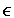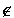Recipient-Hiding and Related Properties for Public Key Encryption
Appendix - formal proofs
```
```
Proof of Lemma 1:
 Aopt.guess(r1, ..., rN) = 1, if riS1 \ S0 for some i1..N = 0, otherwise
This follows from the fact that:
• when one or more of the ri are in S1 \ S0, then b = 1 with probability 1.
• otherwise, b has a slightly greater than 1/2 probability of being 0, but the values of the ri give no additional information about b (so no adversary can do better than guessing b = 0 in this case).
When b = 1:
 Pr[riS1 \ S0 for some i] = 1 - (|S0| / |S1|)N < 1 - (1-u)N < N.u (because (1-u)N > 1 - N.u)
When b = 0:
Pr[riS1 \ S0 for any i] = 1
Therefore Pr[Aopt.guess(S0, S1, N) = b] < (1/2.N.u) + (1/2.1)

 I.e. Adv(Aopt, S0, S1, N) < 2.(1/2.N.u + 1/2) - 1 = N.u

So Adv(A, S0, S1, N) < N.u for any A, because Aopt is optimal.

Proof of Lemma 2:

Proof of security of BRH-DHAES against Chosen Plaintext Attack:

Proof of security of BRH-DHAES against Non-Adaptive Chosen Ciphertext Attack:

Proof of security of BRH-DHAES against Adaptive Chosen Ciphertext Attack:

 David Hopwood